: References : Two dimensional anelastic model : 5 Radiation

# 6 Ground surface

The grand temperature is calculated by the 1D thermal conduction equation.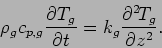(55)

where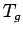is the grand temperature (K),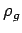is the soil density (kgm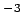),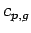is the specific heat of soil (Jkg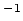K), and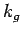is the thermal conductivity (WmK). The surface temperature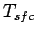is given by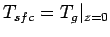.

The boundary condition at the surface is given as follows.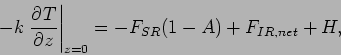(56)

where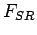is the solar radiative flux at the surface (the sign of downward flux is positive),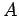is the surface albedo,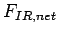is the net infrared radiative flux emitted from the surface and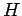is the sensible heat flux (the sign of upward flux is positive). The lower boundary of the grand surface is given as a insulation boundary.

## Parameters

The values of soil density, thermal conductivity and specific heat are same as those of standard model of Kieffer et al. (1977).

 Parameters Standard values Note0.25 Kieffer et al. (1977)1650 kgm$B!7(B588 JKkg$B!7(B7.63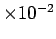JKmsec$B!7(B By using these values, the thermal inertia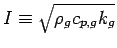is 272 Wm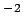sec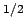Kand the diurnal skin depth of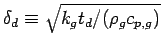is about 8.2 cm.: References : Two dimensional anelastic model : 5 Radiation Odaka Masatsugu $BJ?@.(B19$BG/(B4$B7n(B25\$BF|(B